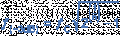# 2nd order HPF cutoff frequency

#### rpschultz

Joined Nov 23, 2022
239
Hi,

Electronics-tutorials has a nice write up on passive LOW pass filters. Within that it shows how the corner frequency changes between a 1st and 2nd order.But I'm interested in passive HIGH pass filters. Same website has a similar article, but it doesn't have a corresponding formula for frequency difference between 1st and 2nd order. I don't think it can be the same formula. With LOW pass the 2nd order cut is lower than 1st order, sharper. But it should be the opposite with HIGH pass... the 2nd order cut should be higher than 1st, right? Maybe just invert the formula?

#### MrAl

Joined Jun 17, 2014
9,630
Hi,

Electronics-tutorials has a nice write up on passive LOW pass filters. Within that it shows how the corner frequency changes between a 1st and 2nd order.
View attachment 282628

But I'm interested in passive HIGH pass filters. Same website has a similar article, but it doesn't have a corresponding formula for frequency difference between 1st and 2nd order. I don't think it can be the same formula. With LOW pass the 2nd order cut is lower than 1st order, sharper. But it should be the opposite with HIGH pass... the 2nd order cut should be higher than 1st, right? Maybe just invert the formula?

Hello there,

Yes, but there is just one, big, big, little, catch.

That formula is not for a passive LP (or inverted, for passive HP) filter. That formula is for a 2nd order filter with a 'pure' 2nd order reponse. That is, where the two stages are completely independent of each other with respect to their impedances. In other words, the two stages are isolated from each other with a buffer stage.
That means if you have one LP or HP filter with R and C and you connect it directly to another LP or HP filter with identical R and C you will not get that ratio. You would have to connect the second RC section with a buffer with a gain of 1, which is a voltage follower. Using the buffer you would see that ratio for LP and the inverse for HP.

Now in the above i mentioned the R's and C's being 'identical'. However, if you make the second section RC with values that are 10 times higher than the first stage, you will get that ratio to some approximation although not that exact ratio. If you make the second stage RC values 100 times higher than the first stage then you will see that ratio to an even better approximation. That's because the second stage impedance has a loading effect on the first stage which alters the response so if you use impedance scaling for the second stage you can get that ratio approximately.
For example if the first stage has R=1k and C=1uf, if the second stage has R=1k and C=1uf you will not see that ratio, but if you make the second stage with R=10k and C=0.1uf you will see that ratio approximately, and if you make the second stage R=100k and C=0.01uf then you will see that ratio to an even better approximation. This is because with each increase in impedance for the second stage we load the first stage less and less and so the response becomes nearly identical to two stages that are isolated with a gain of 1 buffer.

We could develop a formula for non isolated stages also where both R's and C's are the same in both stages. It would be different than the original ratio.

#### rpschultz

Joined Nov 23, 2022
239
Hmmm. Yes I see. Thanks for the response.

#### MrAl

Joined Jun 17, 2014
9,630
Hmmm. Yes I see. Thanks for the response.
Hi,

You're welcome.

Yes if the impedance of the next stage affects the impedance of the previous stage then the ratio changes.

Here is a quick rundown of the way things change when we make the second stage impedance 'a' times the previous stage impedance in a 2nd order filter...

1.553773974030037, perfectly isolated (a=>inf)
1.664600346378735, a=10
1.569314266929646, a=70.8
1.564771999661405, a=100
1.554872771721895, a=1000

So if we used 1k and 1000uf for the first stage, if we used 1Meg and 1uf for the second stage we would get very very close to the same ratio as if we had used a gain of 1 buffer between stages. We see roughly a 1 percent difference with a=70.8 approximately.